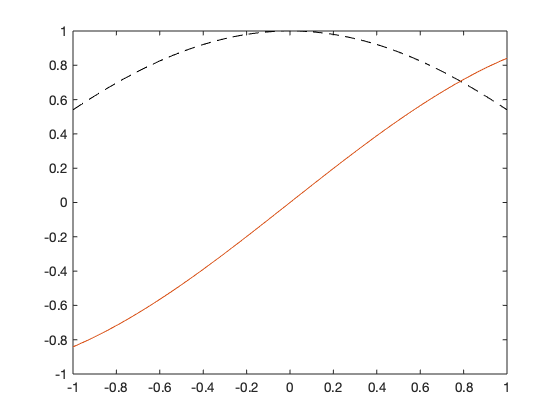# A quick intro to MATLAB¶

## A couple examples from calculus¶

### Limits and continuity

#### Definition¶

A function $~f$ defined on a set $~X$ has limit $~L$ at $~x_0$

$$\lim_{x\to x_0} f(x) = L$$

if, given any real number $\epsilon > 0$, there exists a real number $~\delta > 0$ such that

$$|f(x) - L| < \epsilon, ~~ \text{ whenever } ~~ x \in X ~~ \text{ and } ~~ 0 < |x - x_0| < \delta.$$

Given any real number $\epsilon > 0$, there exists a real number $~\delta > 0$ such that

$$|f(x) - L| < \epsilon, ~~ \text{ whenever } ~~ x \in X ~~ \text{ and } ~~ 0 < |x - x_0| < \delta.$$

If $f(x) = \sin (x)$ and we are given $\epsilon = 10^{-5}$, let's test to see what $\delta$ should be:

f = @(x) sin(x);
epsilon = .00001;
x0 = 0;
x = 0.00001;

format long %display more digits
abs(f(x)-f(x0))
abs(f(x)-f(x0)) < epsilon %returns 0 for false, and 1 for true

ans =

9.999999999833334e-06

ans =

logical

1



#### Definition¶

Let $f$ be a function defined on a set $X$ of real numbers and $x_0 \in X$. Then $f$ is continuous at $x_0$ if

$$\lim_{x \to x_0} f(x) = f(x_0)$$

The set of all continuous functions defined on the set $X$ is denoted by $C(X)$ ($C[a,b]$ or $C(a,b]$ if $X$ is an interval).

The function $f(x) = |x|$ is continuous everywhere but the following function is not

$$g(x) = \begin{cases} -1, & x < 0,\\ 1 & x \geq 0. \end{cases}$$
x = linspace(-1,1,100);
y1 = abs(x);  % evaluate f(x)
y2 = sign(x); % evlauate g(x)
plot(x,y1,'r') % plotted in red
hold on % don't erase the plot for the next plot command
plot(x,y2,'k') % plotted in black
xlabel('x'); ylabel('f(x) and g(x)') %label axes
ylim([-1.1,1.1])# Examples from linear algebra¶

### Vectors¶

v = [1,2,3]; % row vector
w = [1;2;3]; % column vector
v
w

v =

1     2     3

w =

1
2
3


v = [1,2,3]; % row vector
w = [1;2;3]; % column vector
w(1)
v(1:2)
w(2:3)
sum(w)

ans =

1

ans =

1     2

ans =

2
3

ans =

6



### Matrices¶

M = [1,2,3,4;5,6,7,8;9,10,11,12;12,14,15,16]
M(1,4); % get the (1,4) element
M(2:3,2:3);% get the "middle" block of the matrix
M(3,:); % Get row 3
sum(M)

M =

1     2     3     4
5     6     7     8
9    10    11    12
12    14    15    16

ans =

27    32    36    40



### For loop¶

Add all positive integers less $n+1$

n = 10;
S = 0; % using capital letters because sum() is a built-in function
for i = 1:n
S = S + i;
end
S
n*(n+1)/2

S =

55

ans =

55



### While loop¶

Find the power of 2 that is greater than or equal to $n$ but less than $2n$

n = 130;
m = 1;
while m <= n
m = m*2;
end
m

m = 256


### Plotting

x = linspace(-1,1,300); % 300 equally-spaced points between -1 & 1
plot(x,sin(x)) % Plot the sin function
plot(x,cos(x),'--k'); % Plot cos in dashed black, replaces the plot
hold on
plot(x,sin(x)) % Plot together